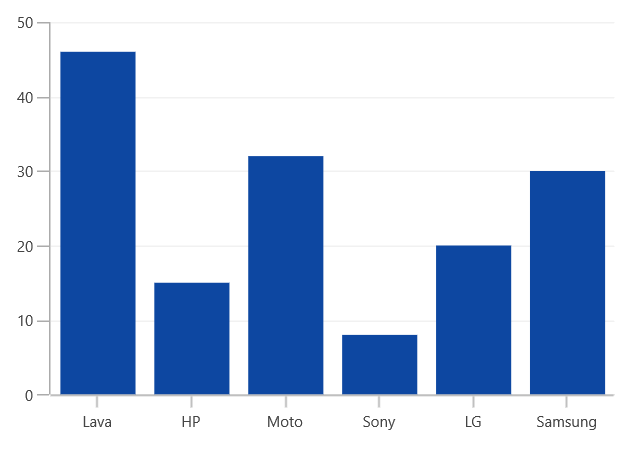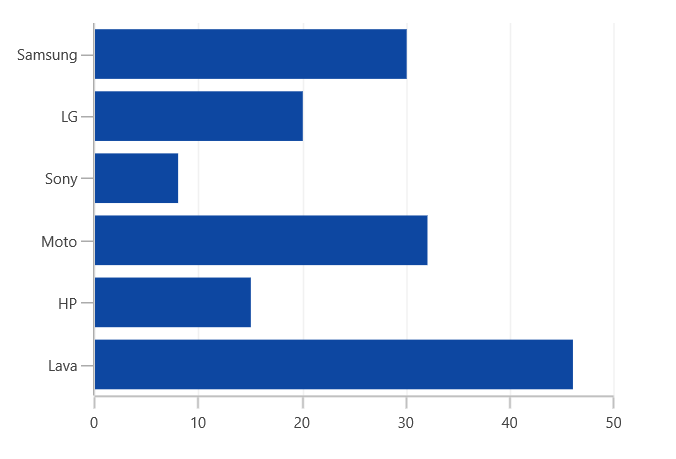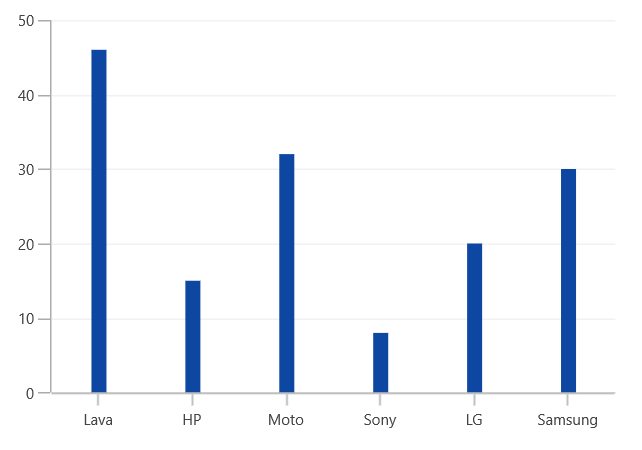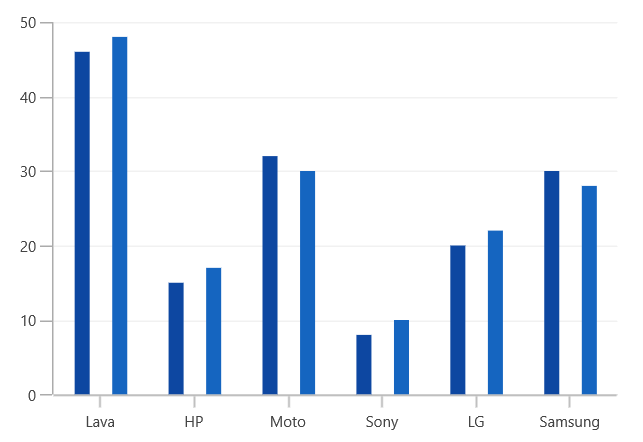# Column and Bar in WinUI Chart

31 May 20222 minutes to read

## Column

Column charts plot discrete rectangles for the given values. The following code example demonstrates the usage of `ColumnSeries`.

``<chart:ColumnSeries ItemsSource="{Binding Data}" XBindingPath="XValue" YBindingPath="YValue"/>``
``````ColumnSeries series = new ColumnSeries()
{

ItemsSource = new ViewModel().Data,

XBindingPath = "XValue",

YBindingPath = "YValue",

};## Bar

Bar series is similar to column series, except the orientation. The following code example shows how to use `BarSeries`.

``<chart:BarSeries ItemsSource="{Binding Data}" XBindingPath="XValue" YBindingPath="YValue"/>``
``````BarSeries series = new BarSeries()
{

ItemsSource = new ViewModel().Data,

XBindingPath = "XValue",

YBindingPath = "YValue",

};``````### Spacing

`Spacing` property of series is used to decide the width of a segment. `Spacing` value ranges from 0 to 1. The following code illustrates how to set `Spacing` property of the series.

``<Chart:ColumnSeries Chart:ChartSeriesBase.Spacing="0.8"/>``
``````ColumnSeries series = new ColumnSeries()

ChartSeriesBase.SetSpacing(series, 0.8);``````Segment Spacing

The `SegmentSpacing` property is used to set the spacing between the segments when multiple series are added in chart. Its value ranges from 0 to 1. The following code illustrates how to use the `SegmentSpacing` property in series.

``````<Chart:SfChart >

<Chart:ColumnSeries SegmentSpacing="0.6"/>

<Chart:ColumnSeries SegmentSpacing="0.6"/>

</Chart:SfChart>``````
``````SfChart chart = new SfChart();

ColumnSeries series1 = new ColumnSeries()
{

SegmentSpacing = 0.6,

};